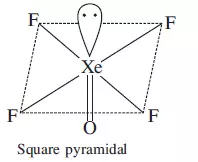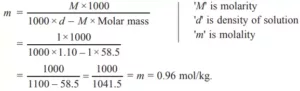# Passage Based Questions Chemistry Class XIPassage Based Questions Chemistry Class XI

The attractive force which holds the two atoms together is called a chemical bond. A covalent bond is formed by an equal sharing of electrons. A coordinate bond is formed by unequal sharing of electrons. An ionic bond is formed by the transfer of electrons from one atom to another. Octet rule, although very useful but it is not universally applicable. According to valence bond theory, a covalent bond is formed by overlapping of half-filled atomic orbitals resulting in lowering of energy and more stability. Bond order is the number of bonds between atoms in a molecule. The higher the bond order more will be stability and bond dissociation enthalpy but smaller bond length. The polarity of a covalent bond depends upon the difference in electronegativity. The covalent character of a bond depends upon polarising power, smaller cation and bigger anions have higher polarising power. VSEPR theory helps to predict the shapes of molecules.

(a) Write the electron dot structure of N2O.
Ans (a). N≡N+ — O

(b) What are the ions present in CsI3?
Ans (b). Cs+ and I3.

(c) Out of CN+, CN, NO, which has the highest bond order?
Ans (c). CN (14): σ(1s)2, σ*(1s)2, σ(2s)2, σ*(2s)2, π(2px)2= π(2py)2, σ (2pz)2.

(d) What is the correct order of repulsion bp – bp, lp – lp, and lp – bp?
Ans (d). lp – lp > lp – bp > bp – bp (bp is bond pair, lp is lone pair).

(e) Draw the structure of XeOF4 on the basis of VSEPR theory.
Ans (e).f) Which out of B2, CO, O22– and NO+ are paramagnetic and why?
Ans (f). B2(10): σ(1s)2, σ*(1s)2, σ(2s)2, σ*(2s)2, π(2px)1= π(2py)1

Passage Based Questions Chemistry Class XI

2. Read the following passage and answer the questions that follow: Modern periodic table arranges the elements in the increasing order of atomic number. It has 18 groups and 7 periods. Atomic numbers are consecutive in a period and increases in the group in a pattern. Elements are divided into four blocks, s-block, p-block, d-block, and f-block based on their electronic configuration. 78% of elements are metals, about 20 elements are non-metals, and few elements like B, Si, Ge, As are metalloids. Metallic character increases down the group but decreases along the period from left to right. The physical and chemical properties vary periodically with their atomic numbers. Periodic trends are observed in atomic size, ionization enthalpies, electron gain enthalpies, electronegativity, and valence. Oxides of metals are basic, some are amphoteric. Non-metals form acidic oxides, some form neutral oxides. s-block elements are soft, highly reactive, do not show variable oxidation states. p-block elements are metals, non-metals as well as metalloids, show variable oxidation states, exist as solids, liquids, and gases. d-block elements are metals, form coloured ions, show variable oxidation states, have high melting and boiling points. Lanthanoids and actinoids are f-block elements, form coloured ions. All actinoids are radioactive.

(a) Name the elements which belong to the d-block but are not transition metals.
Ans (a). Zn, Cd, Hg.

(b) What are representative elements?
Ans (b). s-block and p-block elements are called representative elements or main group elements.

(c) What is the difference between oxidation states of p-block and d-block elements?
Ans (c). In p-block elements, oxidation states differ by 2 and the lower oxidation state is more stable whereas in d-block, oxidation state differ by 1 and mostly higher oxidation state is more stable.

(d) Which group elements are most electropositive and why?
Ans (d). Group 1 due to the largest atomic size and lowest ionization enthalpies in respective periods.

(e) What happens to reactivity down the group 17?
Ans (e). Reactivity goes on decreasing down the group as the tendency to gain electrons decreases.

(f) What type of compounds are formed by elements belonging to group 14 and third period? Identify the element.
Ans (f). Covalent, silicon.

(g) What is the formula of the compound formed between Al and S?
Ans (g). Al2S3

Passage Based Questions Chemistry Class XI

Orbitals are regions or spaces where there is a maximum probability of finding electrons. Qualitatively, these orbitals can be distinguished by their size, shape, and orientation. An orbital of small size means there is more chance of finding the electron near the nucleus. Shape and orientation mean the direction in which the probability of finding the electron is maximum. Atomic orbitals can be distinguished by quantum numbers. Each orbital is designated by three quantum numbers n, l, and ml (magnetic quantum number) which define energy, shape, and orientation but these are not sufficient to explain spectra of multi-electrons atoms. Spin quantum number (ms ) determines the spin of electrons. Spin angular momentum of the electron has two orientations relative to the chosen axis which are distinguished by spin quantum numbers ms which can take values +1/2 and –1/2.

(a) How many orbitals are associated with n = 3?
Ans (a). Number of orbitals = n2 = 32 = 9 orbitals, 3s, 3px , 3py , 3pz , 3dx2-y2, 3dz2 , 3dxy, 3dyz and 3dzx.

(b) Describe the orbitals represented by (i) n = 2, l = 1 (ii) n = 4, l = 0.
Ans (b). (i) 2p (ii) 4s

(c) How many electrons are possible in an orbital? Why?
Ans (c). Orbital can have a maximum of two electrons which must be of opposite spin.

(d) What is the shape of ‘s’ and ‘p’ orbitals?
Ans (d). ‘s’ orbitals are spherical and ‘p’ orbitals have dumb-bell shaped.

(e) Name two d-orbitals that are on-axis.
Ans e. dx2-y2, dz2.

Passage Based Questions Chemistry Class XI

Stoichiometry is a section of chemistry that involves a calculation based on chemical equations. Chemical equations are governed by laws of chemical combination. The mass of reactants is equal to the mass of products. The compound obtained from different methods contains the same elements in the fixed ratio by mass. A mole is a counting unit, equal to 6.022 × 1023 particles. One mole is also equal to molar mass expressed in grams. One mole of every gas at STP has a volume equal to 22.4 L. The reacting species which are consumed in the reaction completely is called limiting reagent which decides the amount of products formed. The concentration of the solution is expressed in terms of molarity, morality, and mole fraction.

(a) Calculate the number of moles of NH3 formed by the reaction of 2 moles of N2 and 2 moles of H2, N2(g) + 3H2(g) → 2NH3(g).
Ans (a). 1 mole of N2 needs 3 moles of H2,
Therefore, 2 moles of N2 needs 6 moles of H2.
But we have only 2 moles of H2, so H2 is a limiting reagent.
3 moles of H2 gives 2 moles of NH3
2 moles of H2 gives 2/3 × 2 = 1.33 moles of NH3.

(b) Calculate number of electrons in 18 g of H2O. [Atomic number of H = 1, O = 8]
Ans (b). 18 g of water (1 mole) containing 10 × 6.022 × 1023 electrons = 6.022 × 1024 electrons.
[1 molecule of H2O = 2 + 8 = 10 e].

(c) Calculate the molality of 1 M NaCl solution having density 1.10 g cm–3. (Molar mass = 58.5 g mol–1)
Ans c. (c).(d) Define mole fraction.
Ans d. It is the ratio of the number of moles of solute (component) to the total number of moles of solute and solvent (all components).

(e) In aqueous solution of glucose xglucose = 0.1, what is xH2O = ?
Ans e. xglucose + xH2O = 1 ⇒ xH2O = 1 – 0.1 = 0.9

Passage Based Questions Chemistry Class XI